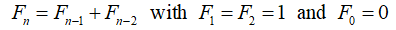# The Fascinating Mathematical Beauty Of The Fibonacci Numbers

• Mulatu Lemma Professor
• Agegnehu Atena
• Tilahun Muche
• Jonathan Lambright
Keywords: Fibonacci numbers, Fibonacci sequences, Pascal’s triangle, Golden ratio

### Abstract

The Fibonacci numbers are sequences of numbers of the form: 0,1,1,2,3,5,8,13,...  Among numerical sequences, the Fibonacci numbers Fn have achieved a kind of celebrity status. These numbers are famous for possessing wonderful and amazing properties. Mathematicians have been fascinated for centuries by the properties and patterns of Fibonacci numbers. In mathematical terms, it is defined by the following recurrence relation:The first number of the sequence is 0, the second number is 1, and each subsequent number is equal to the sum of the previous two numbers of the sequence itself. That is, after two starting values, each number is the sum of the two preceding numbers. In this paper, we give excellent summary of basic properties of Fibonacci numbers as well as and its patterns.  This is a paper which is very helpful for quick reference on Fibonacci numbers.

### Author Biographies

Mulatu Lemma, Professor

Department of Mathematics

College of Science and Technology

Savannah State University USA

Agegnehu Atena

Department of Mathematics College of Science and Technology

Savannah State University Savannah, GA 31404 USA

Tilahun Muche

Department of Mathematics College of Science and Technology
Savannah State University Savannah, GA 31404 USA.

Jonathan Lambright

Department of Mathematics College of Science and Technology
Savannah State University Savannah, GA 31404 USA.

### References

Morris S J, “Fibonacci Numbers”.
http://jwilson.coe.uga.edu/emt669/Student.Folders/Morris.Stephanie/EMT.669/Essay.3/Fibonacci.Essay.html
Knott R, Quinney D A and PASS Maths, ,”The life and numbers of Fibonacci”, 1997
“Fibonacci Number”. Wolfram MathWorld. the web’s most extensive mathematics resource http://mathworld.wolfram.com/FibonacciNumber.html
Spencer D, Invitation To The Number Theory With Pascals.
Burton, D. M., Elementary number theory. New York City, New York: McGraw-Hill. 1998.
Koshy T., Fibonacci and Lucas Numbers with Applications, New York City, New York: John Wiley and Sons, Inc. 2001.
Published
2021-06-13
How to Cite
Lemma, M., Atena, A., Muche, T., & Lambright, J. (2021). The Fascinating Mathematical Beauty Of The Fibonacci Numbers. GPH - International Journal of Mathematics, 4(06), 01-08. Retrieved from http://gphjournal.org/index.php/m/article/view/427
Section
Articles

1 2 > >>